#### Secondary School Sample Questions

Form 1 Form 2 Form 3 Form 4 Form 5Mathematics Form 1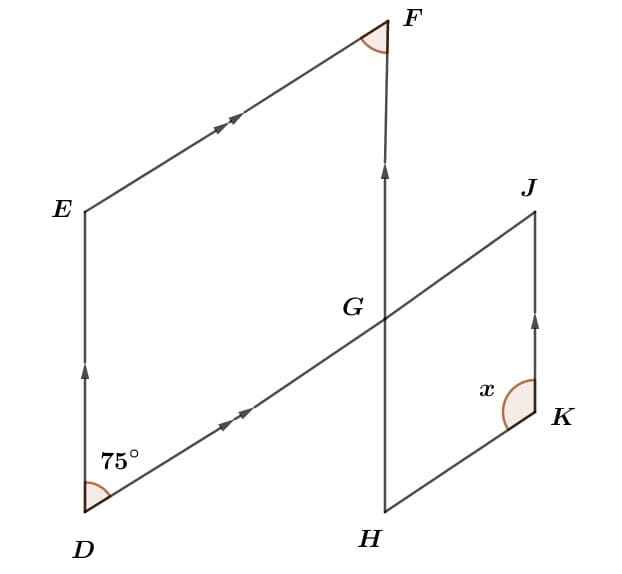The diagram below shows two parallelograms, DEFG and HGJK. DGJ and FGH is the straight line.

Find the value of x.

1. 95°
2. 100°
3. 105°
4. 115°Mathematics Form 2

Simplify $$(3k−1)(3k+1)+$$ $$9 (k+6)^2 \over 2$$

1. $$27\over 2$$$$k^2+54k+161$$

2. $$27\over 2$$$$k^2+54k-161$$

3. $$27\over 2$$$$k^2-54k+161$$

4. $$27\over 2$$$$k^2-54k-161$$Mathematics Form 3

Calculate the value of  $$({4 \over 5})^3$$

1. $$64 \over 125$$

2. $$1 \over 125$$

3. (C)    $$12 \over 125$$

4. $$12 \over 15$$Mathematics Form 5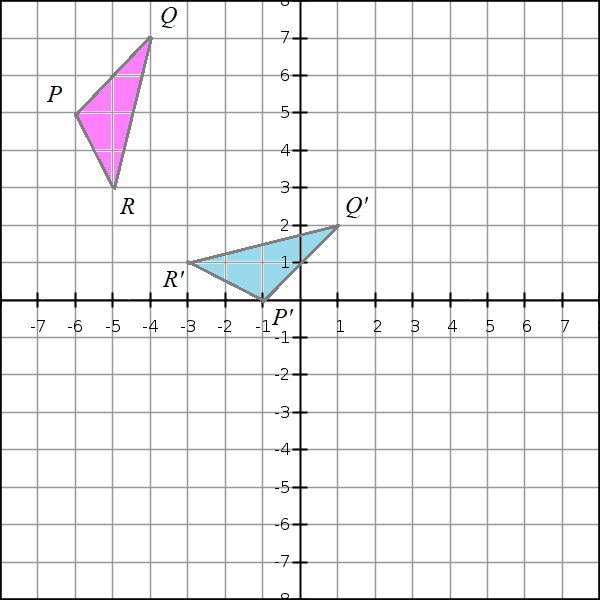Triangle P′Q′R′ in the following diagram is the image of the triangle PQRPQR under a reflection.

Find the axis of reflection.

1. y−x−6=0
2. y−2x+6=0
3. y−x+6=0
4. y−2x−6=0Additional Mathematics Form 4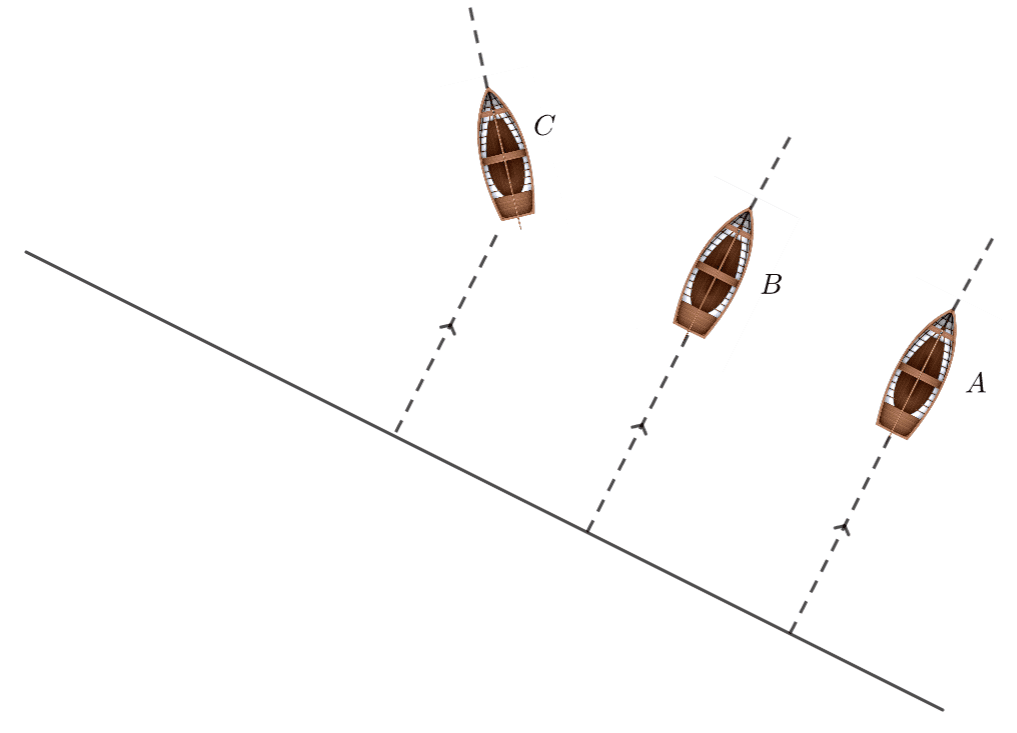The diagram shows the position and the direction of boats A,B and C on a river.

Both boats A and B move in the direction of the water current which flow with a velocity of  $$w= 2i+$$$$1\over 2$$$$j$$    $$ms^-1$$

The velocity of boat A is
$$a=i+j$$     $$ms^-1$$

The velocity of boat B is
$$a=3i+2j$$     $$ms^-1$$

Determine how many times the resultant velocity of boat B compare to the resultant velocity of boat A.

1. $$4\over 3$$

2. $$5\over 3$$

3. $$7\over 3$$

4. $$8\over 3$$Additional Mathematics Form 5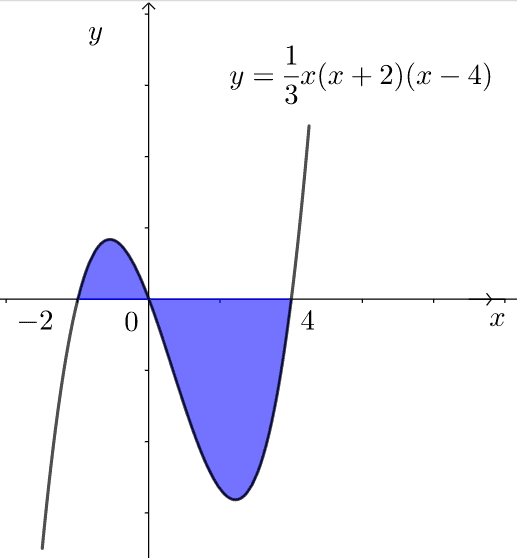The diagram shows the graph of

$$y=$$$$1\over 3$$$$x (x+2)(x−4)$$

Calculate the area of the shaded region.

1. $$148 \over 9$$     $$unit^2$$

2. $$146 \over 9$$     $$unit^2$$

3. $$142 \over 9$$     $$unit^2$$

4. $$140 \over 9$$     $$unit^2$$Physics Form 4

When a signal is transmitted to an object at the bottom of the ocean, a reflection signal is received after 1.5 second. Another signal is reflected to the bottom of the sea and a reflection signal is received after 3 seconds.

If the signal moves in the ocean at a speed of 200 $$ms^-1$$, what is the thickness of the object located at the bottom of the sea?

1. 50   M
2. 1000   M
3. 150   M
4. 200   MPhysics Form 5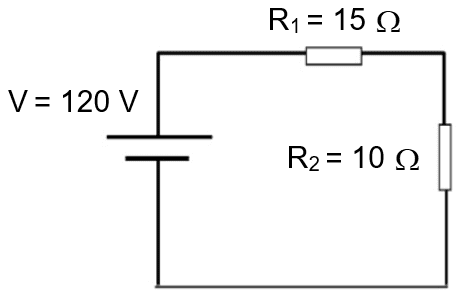The diagram shows an electric circuit.

Determine the effective resistance and current of the circuit.

Effective resistance $$Omega(Ω)$$ Electric current (A)
A 5 4.8
B 25 4.8
C 5 9.5
D 25 9.5Biology Form 4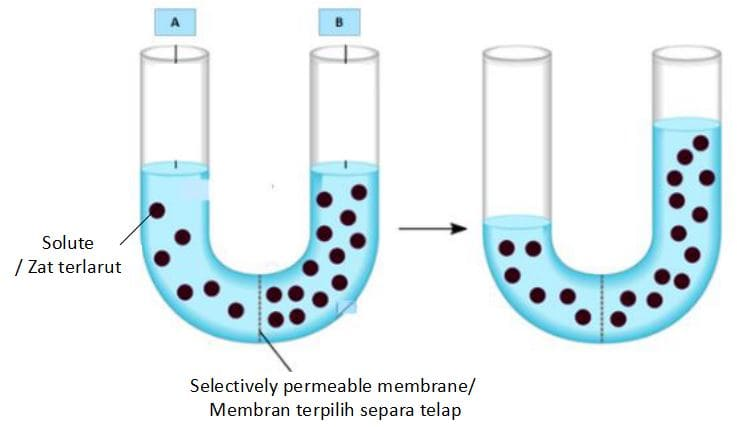The diagram shows the movement of substances across the semi-permeable membrane.

Which of the following is the most precise conclusion at the end of this experiment?

1. The level of solution B rises
2. The level of solution A decreases
3. The water potential in solution A is higher than water potential in solution B
4. Solution A is hypotonic, while, solution B is hypertonicBiology Form 5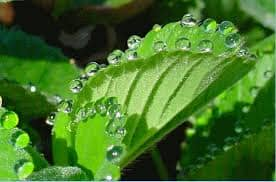The diagram shows a process.

What is the process?

1. Transpiration
2. Photosynthesis
3. Guttation
4. RespirationChemistry Form 4

Calculate the number of oxygen atoms in 2.3 mol of oxygen gas, $$O2$$.

[Avogadro constant,$$Na= 6.02* 10^23$$   $$mol^-1$$]

1. $$2.76 * 10^24$$
2. $$2.76 * 10^23$$
3. $$1.38 * 10^24$$
4. $$1.38 * 10^23$$Chemistry Form 5

Which of the following is the isomer of butanol?

• I. Butan-1-ol
• II. Butan-2-ol
• III. 2-methylpropan-1-ol
• IV. 2-methylpropan-2-ol

1. I, II & III
2. II, III & IV
3. I, III & IV
4. All of the above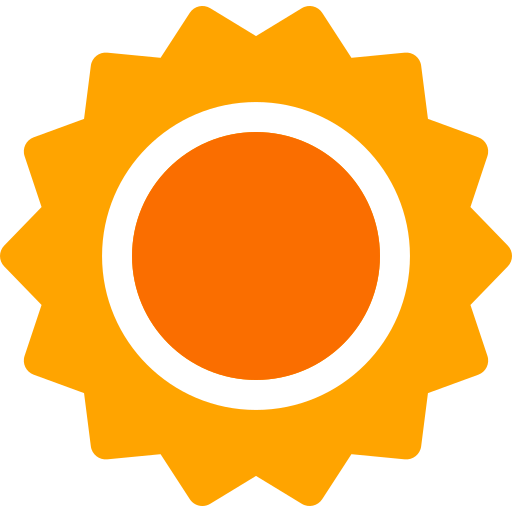Science Form 1

Which of the following are not negative effects of irregular menstruation?

• I. Having difficult in family planning
• II.  Decrease body weight
• III. Has a stable blood content in the body
• IV.   Infertility
1. I & II
2. II & III
3. I, II & III
4. II, III & IVScience Form 4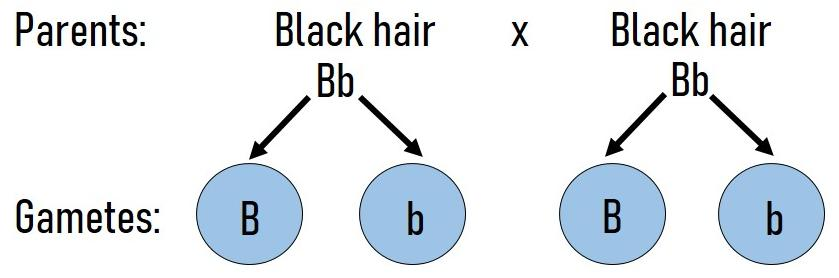The diagram shows a cross-breeding of two traits of humans.

What is the ratio of black hair to brown hair in the first generation?

1. 1 : 3
2. 3 : 1
3. 1 : 1
4. 4 : 0Science Form 5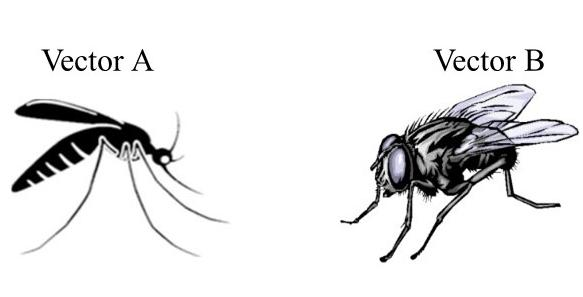The diagram shows vector A and B.

What are the diseases spread by these vectors?

Vector A Vector B
A Tuberculosis Cholera
B Goiter Malaria
C Cholera Dengue fever
D Malaria Cholera